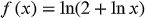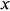1
36
views
56a
Problem

Problem 56a

Chapter 1: Functions and ModelsTextbook ExpertVerified Tutor
14 Nov 2021

Given information

Given function is,Step-by-step explanation

Step 1.

The natural logarithmic function is well defined only for positive real numbers.

So the domain of given function is the set of all values offor which,